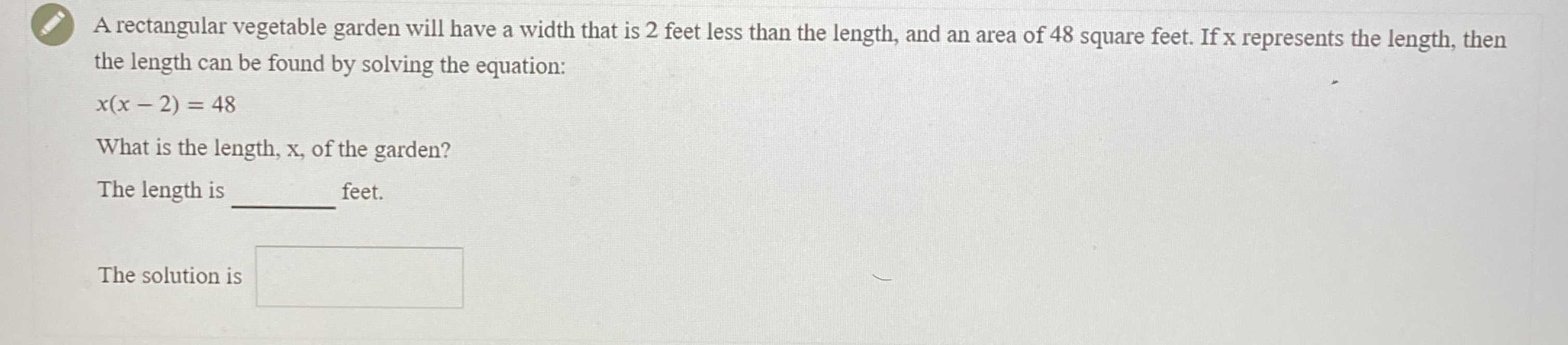### ¿Todavía tienes preguntas de matemáticas?

Pregunte a nuestros tutores expertos
Algebra
Pregunta(2.) A rectangular vegetable garden will have a width that is $$2$$ feet less than the length, and an area of $$48$$ square feet. If $$x$$ represents the length, then the length can be found by solving the equation:

$$x ( x - 2 ) = 48$$

What is the length, $$x$$ , of the garden? The length is feet. The solution is